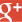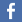﻿ XOR Decrypt & Encrypt with our online tool ﻿

# XOR Online Encrypt & Decrypt## XOR encryption :

The binary operation XOR (stands for eXclusive OR) is a binary operand (as are AND, OR, etc) from Boole algebra. This operand will compare two bits and will produce one bit in return. That bit will be equal to 1 if the two compared bits were different, 0 if they were equal. Xor encryption is commonly used in several symmetric ciphers (especially AES). A symetric cipher is simply a cipher in which the key is used for encryption and decryption process. The XOR operand is so applied to each bit between the text you want to encrypt and the key you'll choose. Examples are better than words, let's take the word "xor". We want to encrypt it with the key "cle". First we have to convert the input and the key in binary representation :

xor : 01111000 01101111 01110010
cle : 01100011 01101100 01100101

Then we compare each bit with the XOR operand. Which will give you this :

xor : 01111000 01101111 01110010
cle : 01100011 01101100 01100101
00011011 00000011 00010111

If we wanted to go back to the original input ("xor"), we would just have to reapply the XOR between the output and the key. You can note that XOR is a commutative function, as multiplications. Now what do we do if the key is smaller than the input ? This is the case in most situations. If the key was the same length than the input, it wouldn't be interesting because you would also have to communicate the key to the person you want reading the input. In contrast, when the key and the input have the same length, it is impossible for someone to crack the cipher. So, when the key is smaller than the input, you just reapply it until you reach the end of input. If by instance I wanted to encrypt the word "bonjour" with my key "cle", I would do it this way :

bonjour : 01100010 01101111 01101110 01101010 01101111 01110101 01110010
cleclec :   01100011 01101100 01100101 01100011 01101100 01100101 01100011

You can see that we simply repeat the key. The smaller you key, the easiest it will be to crack your cipher (obviously). The XOR cracking often are based on frequency analysis. But for that matter, I suggest you to do some researches.

﻿Mentions légales | 24,481,169 cracked hashes since 11/2015 | There are words in the database |